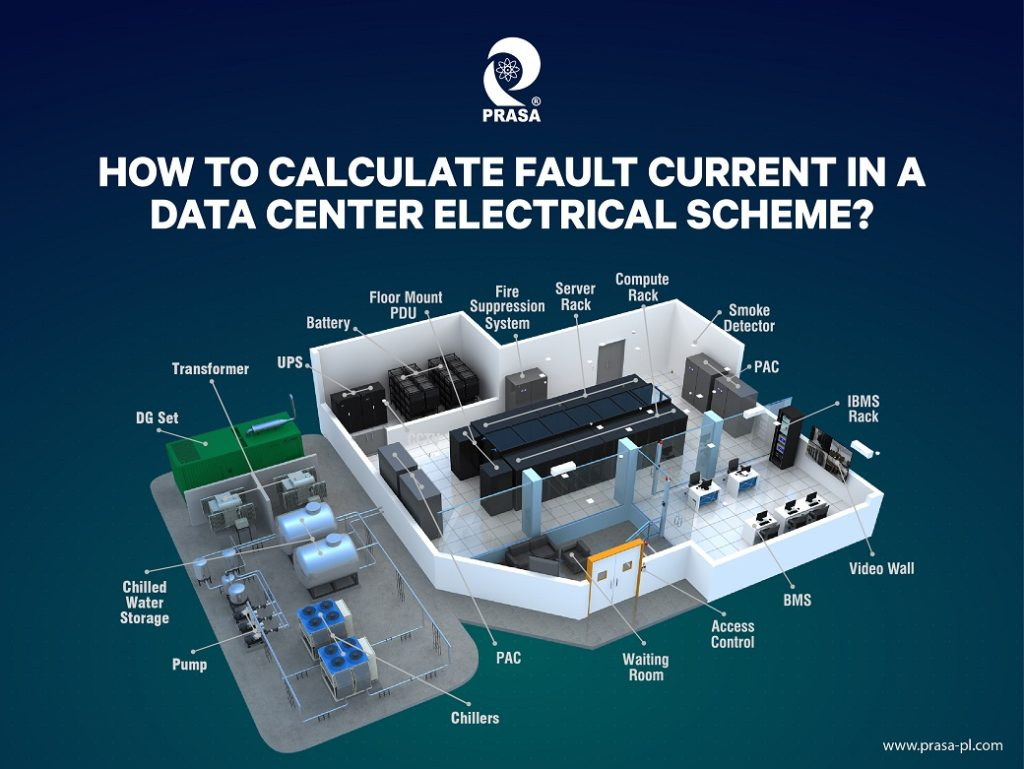## How to calculate fault current in a data center electrical scheme?Calculating fault current in a data center electrical scheme requires an understanding of the electrical components in the system, including the generators, transformers, switchgear, and cables. Here are the general steps for calculating fault current in a data center electrical scheme:

Determine the power source: The fault current calculation begins with identifying the power source, such as a utility grid or a backup generator.

Gather electrical data: Collect electrical data such as the nominal voltage of the system, the available short-circuit current of the power source and the impedance of the electrical components.

Calculate fault current: Using the data collected, you can use the following formula to calculate the available fault current:

Fault Current = Power Source Short Circuit Current / Total Impedance

The total impedance includes the impedance of all electrical components such as cables, transformers, and switchgear.

Analyze the results: Once you have calculated the fault current, you should analyze the results to ensure that the system is properly designed to withstand the expected fault current. This analysis should take into consideration the interrupting rating of the protective devices, such as circuit breakers and fuses.

It is important to note that calculating fault current in a data center electrical scheme is a complex process that requires a thorough understanding of the electrical system and its components. It is recommended that this calculation is performed by a qualified electrical engineer. Additionally, it is essential to regularly perform fault current calculations to ensure that the system is designed to handle any potential faults that may occur.